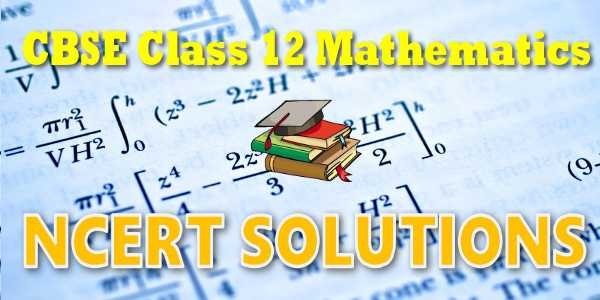# CBSE - Class 12 - Mathematics - Matrices - NCERT Solutions## NEET-UG 2021

10,000+ Daily Practice Questions

(only at ₹ 299/- in myCBSEguide App)

## NCERT Solutions for Mathematics Matrices## myCBSEguide App

Complete Guide for CBSE Students

NCERT Solutions, NCERT Exemplars, Revison Notes, Free Videos, CBSE Papers, MCQ Tests & more.

# NCERT solutions for class 12 Mathematics## NCERT Class 12 Mathematics Chapter-wise Solutions

### Maths Part I

1. Chapter 1 – Relations and Functions
2. Chapter 2 – Inverse Trigonometric Functions
3. Chapter 3 – Matrices
4. Chapter 4 – Determinants
5. Chapter 5 – Continuity and Differentiability
6. Chapter 6 – Application of Derivatives

### Maths Part II

1. Chapter 7 – Integrals
2. Chapter 8 – Application of Integrals
3. Chapter 9 – Differential Equations
4. Chapter 10 – Vector Algebra
5. Chapter 11 – Three Dimensional Geometry
6. Chapter 12 – Linear Programming
7. Chapter 13 – Probability

## CBSE class 12th Mathematics have two books. Each book has chapters and topics.

1. NCERT Mathematics Book Class 12 Part-1
2. NCERT Mathematics Book Class 12 Part-2

Here is the list of topics covered under each chapter of class 12 Mathematics NCERT text book.

### Relations and Functions

• 1.1 Introduction
• 1.2Types of Relations
• 1.3Types of Functions
• 1.4Composition of Functions and Invertible Function
• 1.4Binary Operations

### 2. Inverse Trigonometric Functions

• 2.1 Introduction
• 2.2 Basic Concepts
• 2.3 Properties of Inverse Trigonometric Functions

### 3. Matrices

• 3.1 Introduction
• 3.2 Matrix
• 3.3 Types of Matrices
• 3.4 Operations on Matrices
• 3.5 Transpose of a Matrix
• 3.6 Symmetric and Skew Symmetric Matrices
• 3.7 Elementary Operation (Transformation) of a Matrix
• 8 Invertible Matrices

### 4. Determinants

• 4.1 Introduction
• 4.2 Determinant
• 4.3 Properties of Determinants
• 4.4 Area of a Triangle
• 4.5 Minors and Cofactors
• 4.6 Adjoint and Inverse of a Matrix
• 4.7 Applications of Determinants and Matrices

### 5. Continuity and Differentiability

• 5.1 Introduction
• 5.2 Continuity
• 5.3 Differentiability
• 5.4 Exponential and Logarithmic Functions
• 5.5 Logarithmic Differentiation
• 5.6 Derivatives of Functions in Parametric Forms
• 5.7 Second Order Derivative
• 5.8 Mean Value Theorem

### 6. Application of Derivatives

• 6.1 Introduction
• 6.2 Rate of Change of Quantities
• 6.3 Increasing and Decreasing Functions
• 6.4 Tangents and Normals
• 6.5 Approximations
• 6.6 Maxima and Minima

### 7.Integrals

• 7.1 Introduction
• 7.2 Integration as an Inverse Process of Differentiation
• 7.3 Methods of Integration
• 7.4 Integrals of some Particular Functions
• 7.5 Integration by Partial Fractions
• 7.6 Integration by Parts
• 7.7 Definite Integral
• 7.8 Fundamental Theorem of Calculus
• 7.9 Evaluation of Definite Integrals by Substitution
• 7.10 Some Properties of Definite Integrals

### 8. Application of Integrals

• 8.1 Introduction
• 8.2 Area under Simple Curves

### 9.Differential Equations

• 9.1 Introduction
• 9.2 Basic Concepts

### 10  Vector Algebra

• 10.1 Introduction
• 10.2 Some Basic Concepts
• 10.3 Types of Vectors
• 10.5 Multiplication of a Vector by a Scalar
• 10.6 Product of Two Vectors

### 12.Linear Programming

• 12.1 Introduction
• 12.2 Linear Programming Problem and its Mathematical Formula
• 12.3 Different Types of Linear Programming Problems

### 13. Probability

• 13.1 Introduction
• 13.2 Conditional Probability
• 13.3 Multiplication Theorem on Probability
• 13.4 Independent Events
• 13.5 Bayes' Theorem
• 13.6 Random Variables and its Probability Distributions
• 13.7 Bernoulli Trials and Binomial Distribution

## NCERT Solutions Chapter 3 Matrices

The knowledge of matrices is necessary in various branches of mathematics. Matrices are one of the most powerful tools in mathematics. This mathematical tool simplifies our work to a great extent when compared with other straight forward methods. The evolution of concept of matrices is the result of an attempt to obtain compact and simple methods of solving system of linear equations. Matrices are not only used as a representation of the coefficients in system of linear equations, but utility of matrices far exceeds that use. Matrix notation and operations are used in electronic spreadsheet programs for personal computer, which in turn is used in different areas of business and science like budgeting, sales projection, cost estimation, analysing the results of an experiment etc. Also, many physical operations such as magnification, rotation and reflection through a plane can be represented mathematically by matrices. Matrices are also used in cryptography. This mathematical tool is not only used in certain branches of sciences, but also in genetics, economics, sociology, modern psychology and industrial management.

## NCERT Solutions for Class 12th Mathematics

NCERT Solutions Class 12 Mathematics PDF (Download) Free from myCBSEguide app and myCBSEguide website. Ncert solution class 12 Mathematics includes text book solutions from both part 1 and part 2. NCERT Solutions for CBSE Class 12 Mathematics have total 13 chapters. Class 12 Mathematics ncert Solutions in pdf for free Download are given in this website. Ncert Mathematics class 12 solutions PDF and Mathematics ncert class 12 PDF solutions with latest modifications and as per the latest CBSE syllabus are only available in myCBSEguide.

## NCERT Solutions for Class 3## myCBSEguide

Trusted by 1 Crore+ Students

#### CBSE Test Generator

Create papers in minutes

Print with your name & Logo

3 Lakhs+ Questions

Solutions Included

Based on CBSE Blueprint

Best fit for Schools & Tutors

#### Work from Home

• Work from home with us
• Create questions or review them from home

No software required, no contract to sign. Simply apply as teacher, take eligibility test and start working with us. Required desktop or laptop with internet connection